# Every binomial polynomial is irreducible but not prime in the ring of integer-valued polynomials over rational integers

Jump to: navigation, search

## Statement

In the ring of integer-valued polynomials over rational integers, every binomial polynomial, i.e., every polynomial of the form: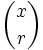$\binom{x}{r}$

is an irreducible element but not a prime element.

## Proof

### The binomial polynomial is irreducible

For this, we need a lemma: the largest number that we can guarantee divides the value of any polynomial of the form$\prod_{i=1}^r (x - a_i)$ for all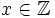$x \in \mathbb{Z}$ is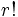$r!$.

With this lemma, we observe that if$\binom{x}{r}$ is expressed as a product of polynomials of smaller degree, each of them is of the form$(p_i/q_i)$ times a product of linear polynomials. In each of the cases, we get that$q_i$ is bounded from above by the factorial of the number of linear polynomials in that factor. Thus, the product of all the$q_i$s is bounded by the product of the factorials, which is strictly less than$r!$, a contradiction.

### The binomial polynomial is not prime

For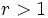$r > 1$, we have:$r!\binom{x}{r} = x(x-1)(x-2) \dots (x-r + 1)$.

This yields: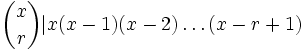$\binom{x}{r} | x(x-1)(x-2) \dots (x-r+1)$.

On the other hand, we have that since$r > 1$,$\binom{x}{r}$ does not divide any of the linear polynomials$x - i$.

For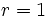$r = 1$, use that: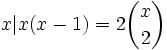$x | x(x-1) = 2\binom{x}{2}$

but$x$ does not divide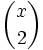$\binom{x}{2}$, since the ratio,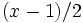$(x-1)/2$, is not an integer-valued polynomial.